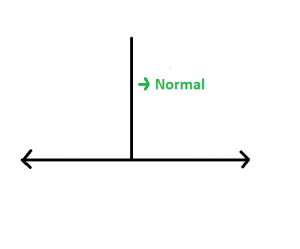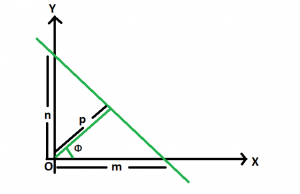GeeksforGeeks App
Open AppBrowser
Continue

# Length of the normal from origin on a straight line whose intercepts are given

Given the intercepts of a straight line on both the axes as m and n. The task is to find the length of the normal on this straight line from the origin.
Examples:

Input: m = 5, n = 3
Output: 2.57248
Input: m = 13, n = 9
Output: 7.39973

Approach: A normal to a line is a line segment drawn from a point perpendicular to the given line.Let p be the length of the normal drawn from the origin to a line, which subtends an angle Θ with the positive direction of x-axis as follows.Then, we have cos Θ = p / m and sin Θ = p / n
Since, sin2 Θ + cos2 Θ = 1
So, (p / m)2 + (p / n)2 = 1
We get, p = m * n / √(m2 + n2)
Below is the implementation of the above approach:

## C++

 `// C++ implementation of the approach``#include ``using` `namespace` `std;` `// Function to find the normal``// of the straight line``float` `normal(``float` `m, ``float` `n)``{``    ``// Length of the normal``    ``float` `N = (fabsf(m) * fabsf(n))``              ``/ ``sqrt``((fabsf(m) * fabsf(m))``                     ``+ (fabsf(n) * fabsf(n)));` `    ``return` `N;``}` `// Driver code``int` `main()``{``    ``float` `m = -5, n = 3;``    ``cout << normal(m, n);` `    ``return` `0;``}`

## Java

 `// Java implementation of the approach``class` `GFG``{` `// Function to find the normal``// of the straight line``static` `float` `normal(``float` `m, ``float` `n)``{``    ``// Length of the normal``    ``float` `N = (``float``) ((Math.abs(m) * Math.abs(n))``            ``/ Math.sqrt((Math.abs(m) * Math.abs(m))``                    ``+ (Math.abs(n) * Math.abs(n))));` `    ``return` `N;``}` `// Driver code``public` `static` `void` `main(String[] args)``{``    ``float` `m = -``5``, n = ``3``;``    ``System.out.println(normal(m, n));``}``}` `// This code has been contributed by 29AjayKumar`

## Python3

 `# Python3 implementation of the approach``import` `math;` `# Function to find the normal``# of the straight line``def` `normal(m, n):` `    ``# Length of the normal``    ``N ``=` `((``abs``(m) ``*` `abs``(n)) ``/``        ``math.sqrt((``abs``(m) ``*` `abs``(m)) ``+``                  ``(``abs``(n) ``*` `abs``(n))));` `    ``return` `N;` `# Driver code``m ``=` `-``5``; n ``=` `3``;``print``(normal(m, n));` `# This code is contributed``# by Akanksha Rai`

## C#

 `// C# implementation of the approach``using` `System;` `class` `GFG``{` `// Function to find the normal``// of the straight line``static` `float` `normal(``float` `m, ``float` `n)``{``    ``// Length of the normal``    ``float` `N = (``float``)((Math.Abs(m) * Math.Abs(n)) /``                       ``Math.Sqrt((Math.Abs(m) * Math.Abs(m)) +``                                 ``(Math.Abs(n) * Math.Abs(n))));` `    ``return` `N;``}` `// Driver code``public` `static` `void` `Main()``{``    ``float` `m = -5, n = 3;``    ``Console.Write(normal(m, n));``}``}` `// This code is contributed by Akanksha Rai`

## PHP

 ``

## Javascript

 ``

Output:

`2.57248`

Time Complexity: O(1)
Auxiliary Space: O(1)

My Personal Notes arrow_drop_up